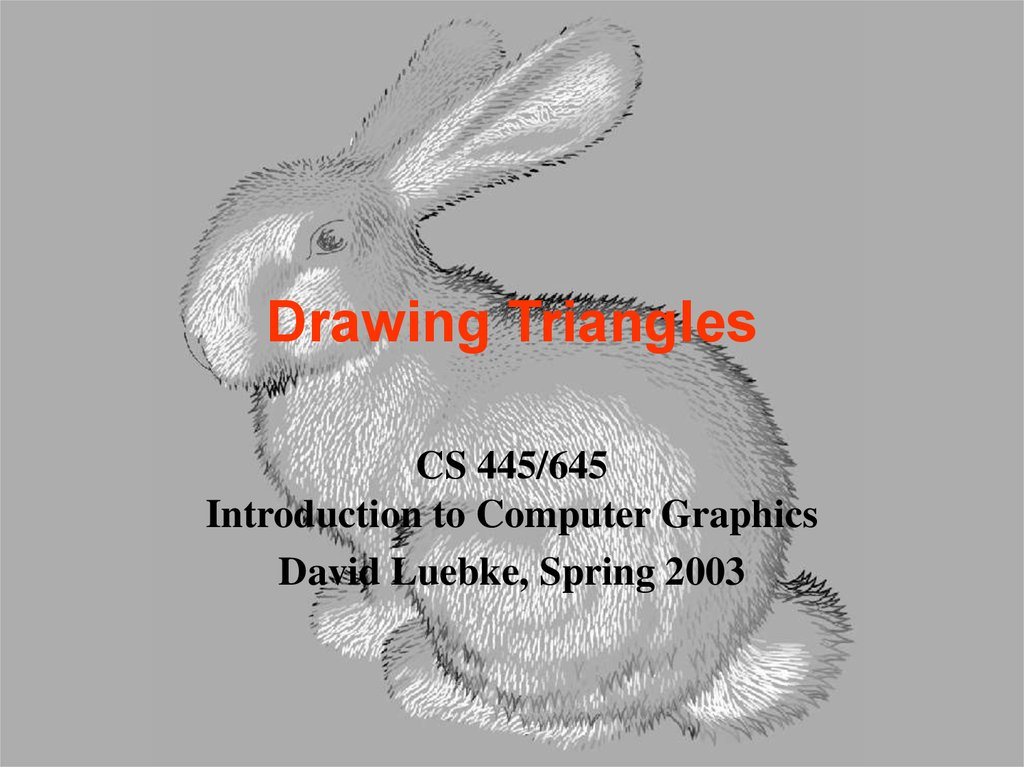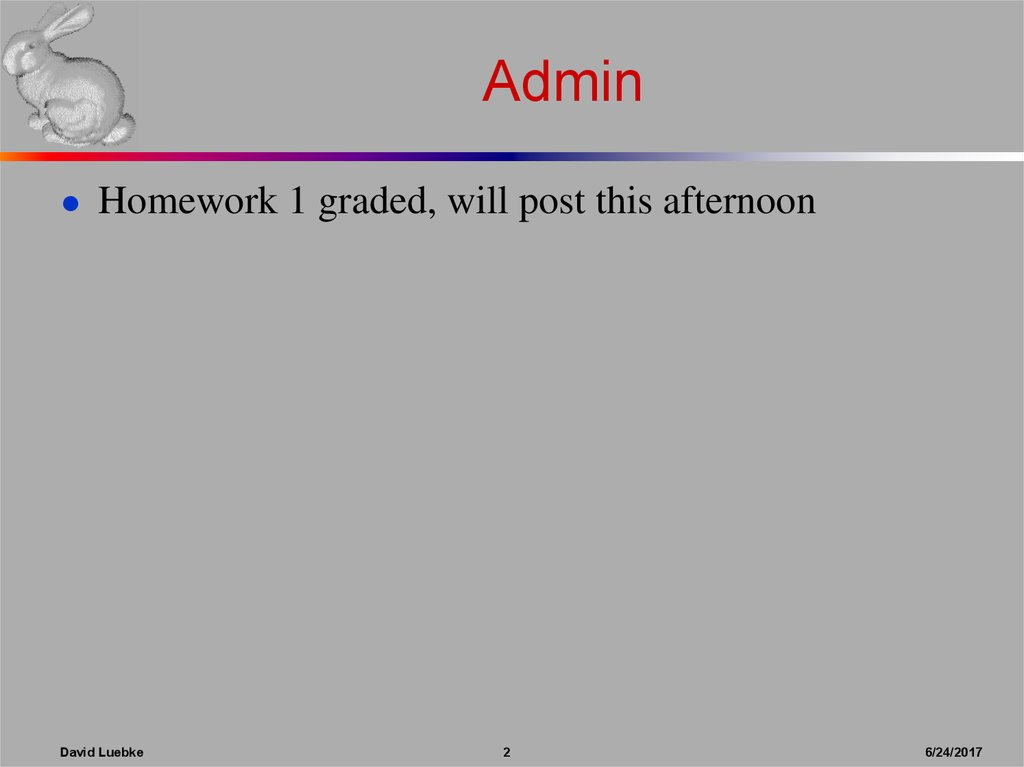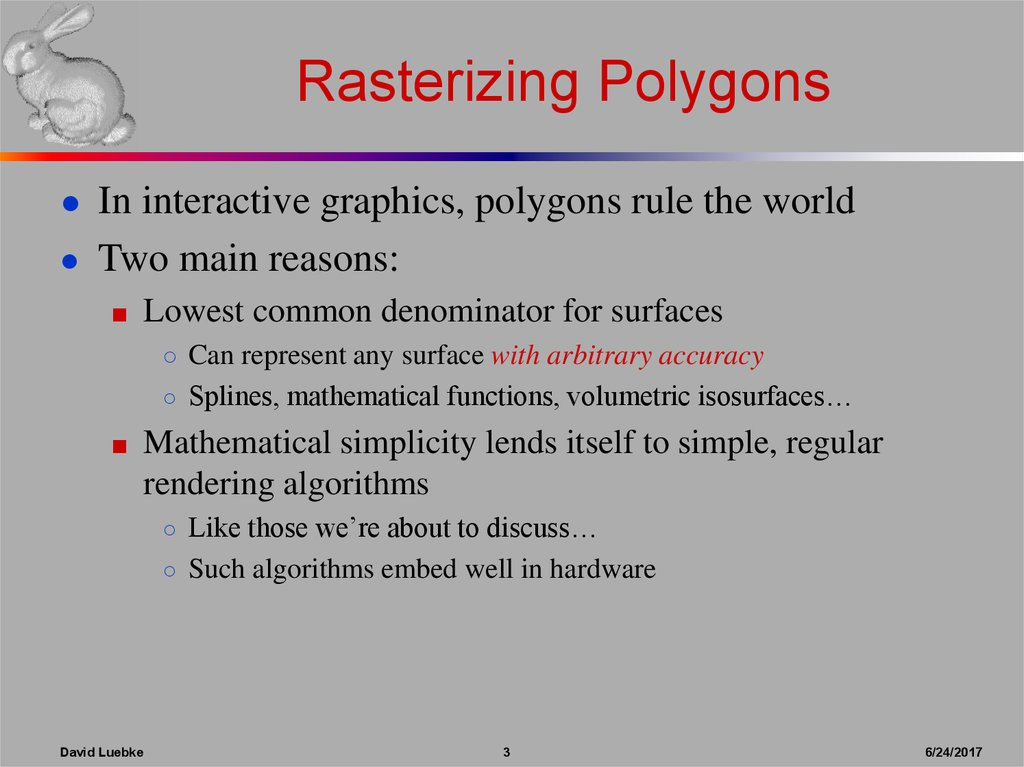Drawing triangles

1. Drawing Triangles

CS 445/645
Introduction to Computer Graphics
David Luebke, Spring 2003

● Homework 1 graded, will post this afternoon
David Luebke
2
6/24/2017

3. Rasterizing Polygons

● In interactive graphics, polygons rule the world
● Two main reasons:
■ Lowest common denominator for surfaces
○ Can represent any surface with arbitrary accuracy
○ Splines, mathematical functions, volumetric isosurfaces…
■ Mathematical simplicity lends itself to simple, regular
rendering algorithms
○ Like those we’re about to discuss…
○ Such algorithms embed well in hardware
David Luebke
3
6/24/2017

4. Rasterizing Polygons

● Triangle is the minimal unit of a polygon
■ All polygons can be broken up into triangles
○ Convex, concave, complex
■ Triangles are guaranteed to be:
○ Planar
○ Convex
■ What exactly does it mean to be convex?
David Luebke
4
6/24/2017

5. Convex Shapes

● A two-dimensional shape is convex if and only if
every line segment connecting two points on the
boundary is entirely contained.
David Luebke
5
6/24/2017

6. Convex Shapes

● Why do we want convex shapes for rasterization?
● One good answer: because any scan line is
guaranteed to contain at most one segment or span of
a triangle
■ Another answer coming up later
■ Note: Can also use an algorithm which handles concave
polygons. It is more complex than what we’ll present here!
David Luebke
6
6/24/2017

7. Decomposing Polys Into Tris

● Any convex polygon can be trivially decomposed
into triangles
■ Draw it
● Any concave or complex polygon can be decomposed
into triangles, too
■ Non-trivial!
David Luebke
7
6/24/2017

8. Rasterizing Triangles

● Interactive graphics hardware commonly uses edge
walking or edge equation techniques for rasterizing
triangles
● Two techniques we won’t talk about much:
■ Recursive subdivision of primitive into micropolygons
(REYES, Renderman)
■ Recursive subdivision of screen (Warnock)
David Luebke
8
6/24/2017

David Luebke
9
6/24/2017

David Luebke
10
6/24/2017

11. Edge Walking

● Basic idea:
■ Draw edges vertically
■ Fill in horizontal spans for each scanline
■ Interpolate colors down edges
■ At each scanline, interpolate
edge colors across span
David Luebke
11
6/24/2017

12. Edge Walking: Notes

● Order vertices in x and y
■ 3 cases: break left, break right, no break
● Walk down left and right edges
■ Fill each span
■ Until breakpoint or bottom vertex is reached
■ Lots of finicky special cases
■ Tough to get right
■ Need to pay attention to fractional offsets
David Luebke
12
6/24/2017

13. Edge Walking: Notes

● Fractional offsets:
● Be careful when interpolating color values!
● Also: beware gaps between adjacent edges
David Luebke
13
6/24/2017

14. Edge Equations

● An edge equation is simply the equation of the line
containing that edge
■ Q: What is the equation of a 2D line?
■ A: Ax + By + C = 0
■ Q: Given a point (x,y), what does plugging x & y into this
equation tell us?
■ A: Whether the point is:
○ On the line: Ax + By + C = 0
○ “Above” the line: Ax + By + C > 0
○ “Below” the line: Ax + By + C < 0
David Luebke
14
6/24/2017

15. Edge Equations

● Edge equations thus define two half-spaces:
David Luebke
15
6/24/2017

16. Edge Equations

● And a triangle can be defined as the intersection of
three positive half-spaces:
David Luebke
16
6/24/2017

17. Edge Equations

● So…simply turn on those pixels for which all edge
equations evaluate to > 0:
-+ +
+David Luebke
17
6/24/2017

18. Using Edge Equations

● An aside: How do you suppose edge equations are
implemented in hardware?
● How would you implement an edge-equation
rasterizer in software?
■ Which pixels do you consider?
■ How do you compute the edge equations?
■ How do you orient them correctly?
David Luebke
18
6/24/2017

19. Using Edge Equations

● Which pixels: compute min,max bounding box
● Edge equations: compute from vertices
● Orientation: ensure area is positive (why?)
David Luebke
19
6/24/2017

20. Computing a Bounding Box

● Easy to do
● Surprising number of speed hacks possible
■ See McMillan’s Java code for an example
David Luebke
20
6/24/2017

21. Computing Edge Equations

● Want to calculate A, B, C for each edge from (xi, yi)
and (xj, yj)
● Treat it as a linear system:
Ax1 + By1 + C = 0
Ax2 + By2 + C = 0
● Notice: two equations, three unknowns
● Does this make sense? What can we solve?
● Goal: solve for A & B in terms of C
David Luebke
21
6/24/2017

22. Computing Edge Equations

x0
x1
● Set up the linear system:
A
y1 y 0
C
B x0 y1 x1 y 0 x1 x 0
● Multiply both sides
by matrix inverse:
● Let C
= x0 y1 - x1 y0 for convenience
■ Then
David Luebke
y 0 A
1
C
y1 B
1
A = y0 - y1 and B = x1 - x0
22
6/24/2017

23. Computing Edge Equations: Numerical Issues

● Calculating C
= x0 y1 - x1 y0 involves some
numerical precision issues
■ When is it bad to subtract two floating-point numbers?
■ A: When they are of similar magnitude
○ Example: 1.234x104 - 1.233x104 = 1.000x101
○ We lose most of the significant digits in result
■ In general, (x0,y0) and (x1,y1) (corner vertices of a triangle)
are fairly close, so we have a problem
David Luebke
23
6/24/2017

24. Computing Edge Equations: Numerical Issues

● We can avoid the problem in this case by using our
definitions of A and B:
A = y0 - y1
B = x 1 - x0
Thus:
C = -Ax0 - By0
or
● Why is this better?
● Which should we choose?
C = x0 y1 - x1 y0
C = -Ax1 - By1
■ Trick question! Average the two to avoid bias:
C = -[A(x0+x1) + B(y0+y1)] / 2
David Luebke
24
6/24/2017

25. Edge Equations

● So…we can find edge equation from two verts.
● Given three corners C0, C1, C0 of a triangle, what
are our three edges?
How do we make sure the half-spaces defined by the
edge equations all share the same sign on the
interior of the triangle?
A: Be consistent (Ex: [C0 C1], [C1 C2], [C2 C0])
How do we make sure that sign is positive?
A: Test, and flip if needed (A= -A, B= -B, C= -C)
David Luebke
25
6/24/2017

26. Edge Equations: Code

● Basic structure of code:
■ Setup: compute edge equations, bounding box
■ (Outer loop) For each scanline in bounding box...
■ (Inner loop) …check each pixel on scanline, evaluating
edge equations and drawing the pixel if all three are
positive
David Luebke
26
6/24/2017

27. Optimize This!

findBoundingBox(&xmin, &xmax, &ymin, &ymax);
setupEdges (&a0,&b0,&c0,&a1,&b1,&c1,&a2,&b2,&c2);
/* Optimize this: */
for (int y = yMin; y <= yMax; y++) {
for (int x = xMin; x <= xMax; x++) {
float e0 = a0*x + b0*y + c0;
float e1 = a1*x + b1*y + c1;
float e2 = a2*x + b2*y + c2;
if (e0 > 0 && e1 > 0 && e2 > 0)
setPixel(x,y);
}
}
David Luebke
27
6/24/2017

28. Edge Equations: Speed Hacks

● Some speed hacks for the inner loop:
int xflag = 0;
for (int x = xMin; x <= xMax; x++) {
if (e0|e1|e2 > 0) {
setPixel(x,y);
xflag++;
} else if (xflag != 0) break;
e0 += a0; e1 += a1; e2 += a2;
}
■ Incremental update of edge equation values
(think DDA)
■ Early termination (why does this work?)
■ Faster test of equation values
David Luebke
28
6/24/2017

29. Edge Equations: Interpolating Color

● Given colors (and later, other parameters) at the
vertices, how to interpolate across?
● Idea: triangles are planar in any space:
■ This is the “redness”
parameter space
■ Note:plane follows form
z = Ax + By + C
■ Look familiar?
David Luebke
29
6/24/2017

30. Edge Equations: Interpolating Color

● Given redness at the 3 vertices, set up the linear
system of equations:
● The solution works out to:
David Luebke
30
6/24/2017

31. Edge Equations: Interpolating Color

● Notice that the columns in the matrix are exactly
the coefficients of the edge equations!
● So the setup cost per parameter is basically a matrix
multiply
● Per-pixel cost (the inner loop) cost equates to
tracking another edge equation value
David Luebke
31
6/24/2017

32. Triangle Rasterization Issues

● Exactly which pixels should be lit?
● A: Those pixels inside the triangle edges
● What about pixels exactly on the edge? (Ex.)
■ Draw them: order of triangles matters (it shouldn’t)
■ Don’t draw them: gaps possible between triangles
● We need a consistent (if arbitrary) rule
■ Example: draw pixels on left or top edge, but not on
right or bottom edge
David Luebke
32
6/24/2017